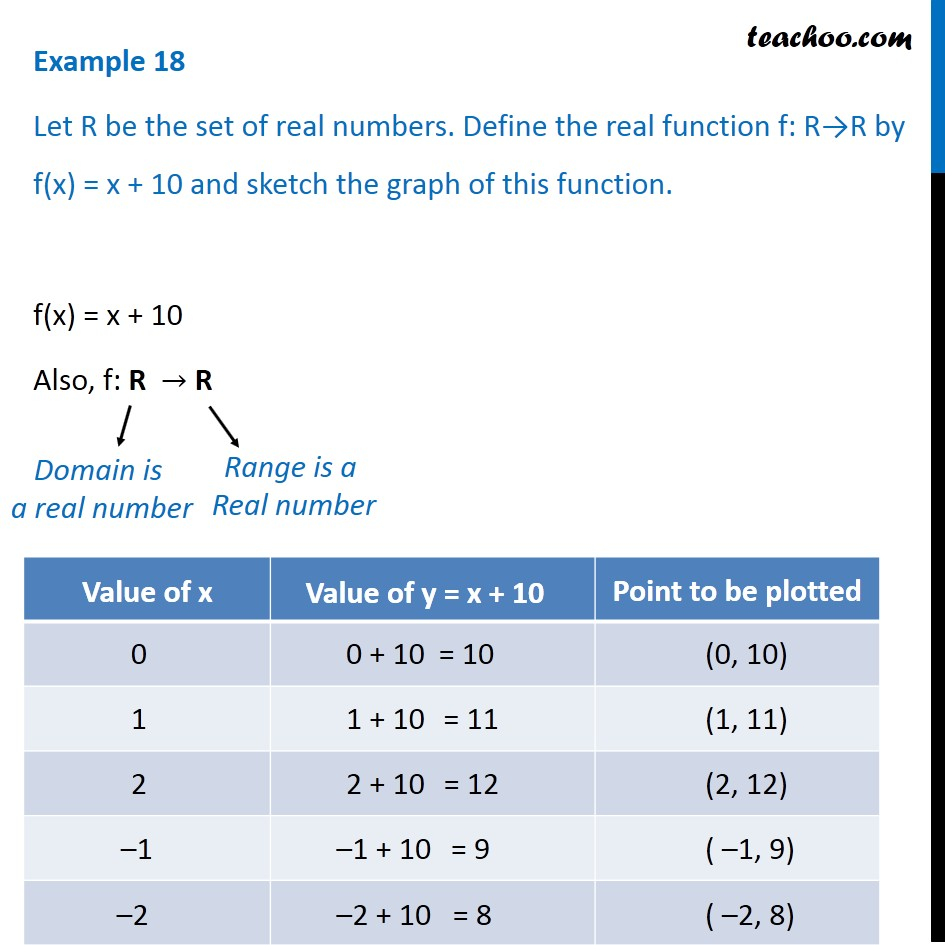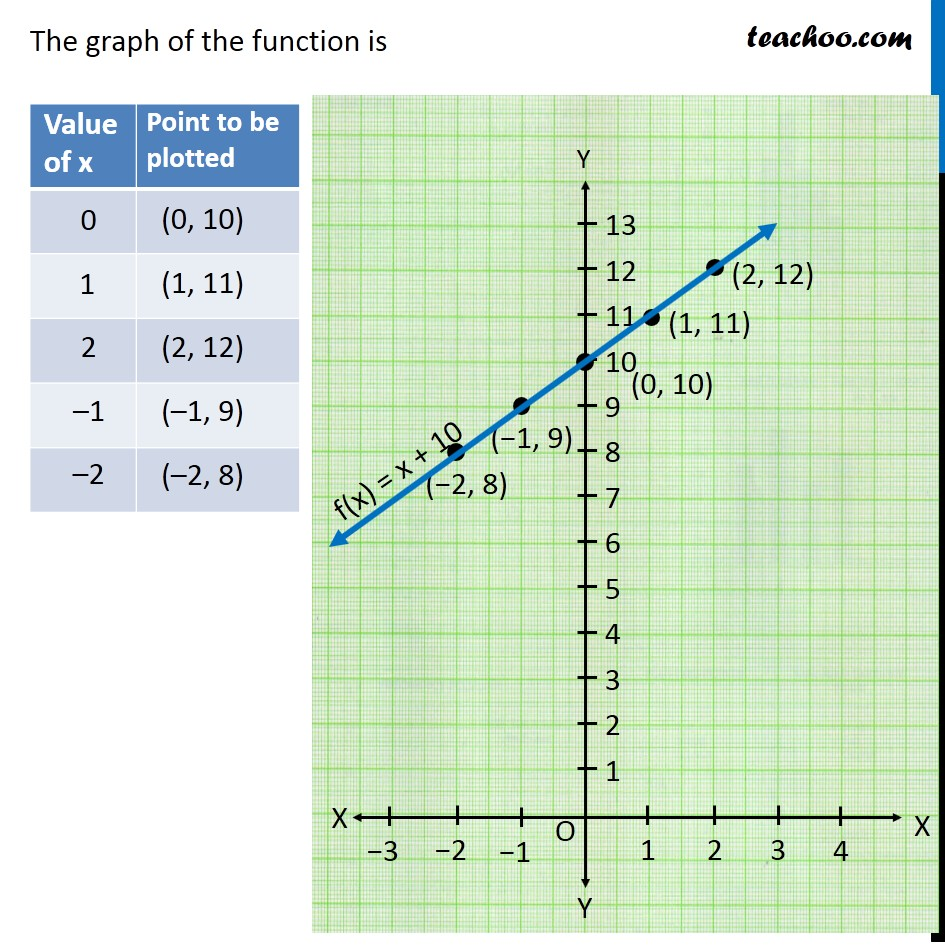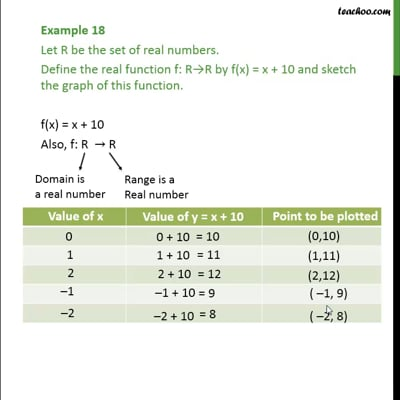Examples

Chapter 2 Class 11 Relations and Functions
Serial order wiseThis video is only available for Teachoo black users

Maths Crash Course - Live lectures + all videos + Real time Doubt solving!

### Transcript

Example 18 Let R be the set of real numbers. Define the real function f: R→R by f(x) = x + 10 and sketch the graph of this function. f(x) = x + 10 Also, f: R → R Domain is a real number Range is a Real number The graph of the function is